## Square Roots and Cube Roots

Note: Square roots and cube roots and operations with them are often included in algebra books.

To find the square root of a number, you want to find some number that when multiplied by itself gives you the original number. In other words, to find the square root of 25, you want to find the number that when multiplied by itself gives you 25. The square root of 25, then, is 5. The symbol for the square root is. Following is a partial list of perfect (whole number) square roots.Note: If no sign (or a positive sign) is placed in front of the square root, the positive answer is required. No sign means that a positive is understood. Only if a negative sign is in front of the square root is the negative answer required. This notation is used in many texts, as well as this book. Therefore,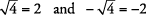To find the cube root of a number, you want to find some number that when multiplied by itself twice gives you the original number. In other words, to find the cube root of 8, you want to find the number that when multiplied by itself twice gives you 8. The cube root of 8, then, is 2 because 2 × 2 × 2 = 8. Notice that the symbol for cube root is the radical sign with a small three (called the index) above and to the left,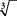. Other roots are similarly defined and identified by the index given. (In square root, an index of 2 is understood and is usually not written.) Following is a partial list of perfect (whole number) cube roots.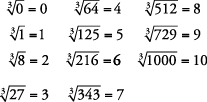To find the square root of a number that is not a perfect square, it is necessary to find an approximate answer by using the procedure given in Example .

Approximate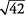.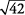is betweenand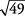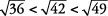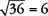andTherefore,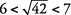Since 42 is almost halfway between 36 and 49,is almost halfway betweenand. Sois approximately 6.5. To check, multiply the following:

6.5 × 6.5 = 42.25 or about 42.

Approximate.Since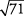is slightly closer tothan it is to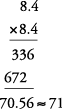Approximate.

First, perform the operation under the radical.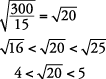Sinceis slightly closer to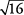than it is to.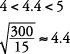Square roots of nonperfect squares can be approximated, looked up in tables, or found by using a calculator. You may want to keep these two in mind, because they are commonly used.Sometimes you will have to simplify square roots, or write them in simplest form. In fractions,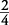can be simplified to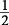. In square roots,can be simplified to.

There are two main methods to simplify a square root.

Method 1:

Factor the number under the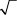into two factors, one of which is the largest possible perfect square. (Perfect squares are 1, 4, 9, 16, 25, 36, 49, and so on)

Method 2:

Completely factor the number under the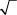into prime factors and then simplify by bringing out any factors that came in pairs.

Simplify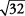.

Method 1.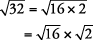Take the square root of the perfect square number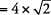Finally, write it as a single expression.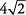Method 2.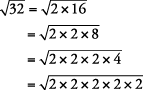Rewrite with pairs under the radicalIn Example , the largest perfect square is easy to see, and Method 1 probably is a faster method.

Simplify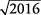.

Method 1.Method 2.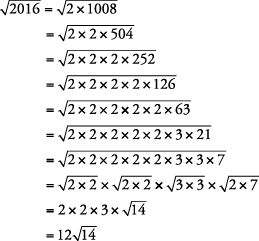In Example , it is not so obvious that the largest perfect square is 144, so method 2 is probably the faster method.

Simplify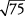.

Method 1.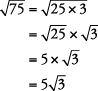Method 2.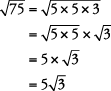Remember: Most square roots cannot be simplified since they are already in simplest form, such as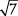.